# SAT II Math II : Midpoint Formula

## Example Questions

### Example Question #1 : Midpoint Formula

Find the point halfway between points A and B.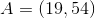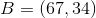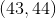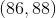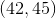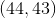Explanation:

Find the point halfway between points A and B.We are going to need to use midpoint formula. If you ever have difficulty recalling midpoint formula, try to recall that it is basically taking two averages. One average is the average of your x values, the other average is the average of your y values.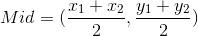Now we plug and chug!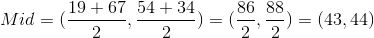### Example Question #2 : Midpoint Formula

What is the coordinates of the point exactly half way between (-2, -3) and (5, 7)?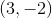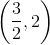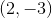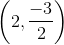Explanation:

We need to use the midpoint formula to solve this question.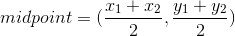In our case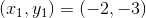and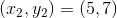Therefore, substituting these values in we get the following: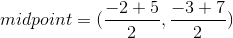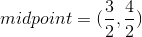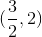### Example Question #3 : Midpoint Formula

Find the midpoint between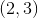and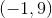.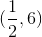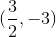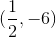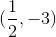Explanation:

Write the midpoint formula.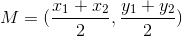Substitute the points.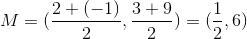The answer is: